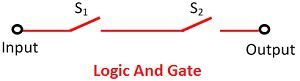# AND Operation

The three Binary Operations are AND Operation, OR and NOT Operation. A binary logic deals with variables having distinct values like true or false, yes or no, hot or cold, high or low, etc.

In a digital system design, assigning the values 1 or 0 to these two values of the variables because digital algebraic operations involve the binary number system.

Binary logics consist of binary variables such as x, y, z, a, b, c, etc. and three basic logical operations namely AND, OR and NOT. Each binary variable is having two and only two distinct values 0 and 1.

Here we are discussing the AND operation. The AND operation is represented by a dot or by the absence of an operator. The And Operation is mostly used in the logical operation in digital electronics and for designing the digital circuit. The symbolic representation of the AND gate is shown below:For example: x.y = z or xy = z and it is read as “x and y is equal to z”. The result of logic operations can be best demonstrated by a truth table.

The truth table for the AND operation is shown below:

xyz
000
010
100
111

The truth table for AND operation shows that when x and y both are zero the output z is also zero. When x is equal to 0 and y is equal to 1 the value of z is 0. When x is equal to 1 and y is equal to 0 then the output z is 0. Similarly, when x and y both is 1, the value of z is also 1.

Also See: OR Operation

Also See: NOT Operation